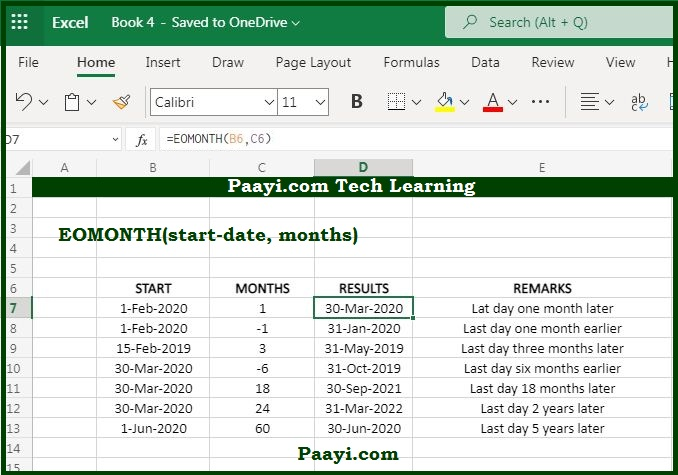# Learn How to Use Microsoft Excel EOMONTH Function

Written by | 0 Comments | 615 Views

In this article, you will learn how to use the Microsoft Excel EOMONTH function and its prime function in Microsoft Excel. You will also get to know the Microsoft Excel EOMONTH function return value and syntax with the help of some examples.

## Microsoft Excel EOMONTH Function

The primary use of the EOMONTH function to get the last day of month n months in the future or past. That means the Microsoft Excel EOMONTH function returns the value containing the last day of the month, n months in the past and future. With the EOMONTH function, you can calculate the expiration dates, due dates, and other dates which are falling on the last day of the month. You can use the positive value to get the future date, while with a negative value, you can get the past date.

### EOMONTH Function Return Value

You can return on the last day of the month.

EOMONTH Function Syntax

= EOMONTH(start-date, months)

Where:

• start-date: The valid Excel serial number format start date.
• months: The count of months before or after the start date.

## How to Use Microsoft Excel EOMONTH Function?As you know, the EOMONTH function is used to get the last day of the month, n months of the past and future. You can get the future date by using the positive value, while you can get the past date by using a negative value for months. With the help of the EOMONTH function, you can calculate the due dates which are falling on the last day of the month. Let's see the usage of the EOMONTH function as given below:

Example1: Let's say the date in cell B1 is May 31, 2020, you can use the EOMONTH as given below:

• = EOMONTH(B1, 0) - Returns the date May 31, 2020
• = EOMONTH(B1, 3) - Return the date August 31, 2020
• =EOMONTH(B1, -1) - Returns the date April 30, 2020

Example 2: You can use the EOMONTH to move through years also:

• = EOMONTH(B1, 12) - Returns the date May 31, 2021
• = EOMONTH(B1, -24) - Returns the date May 31, 2018